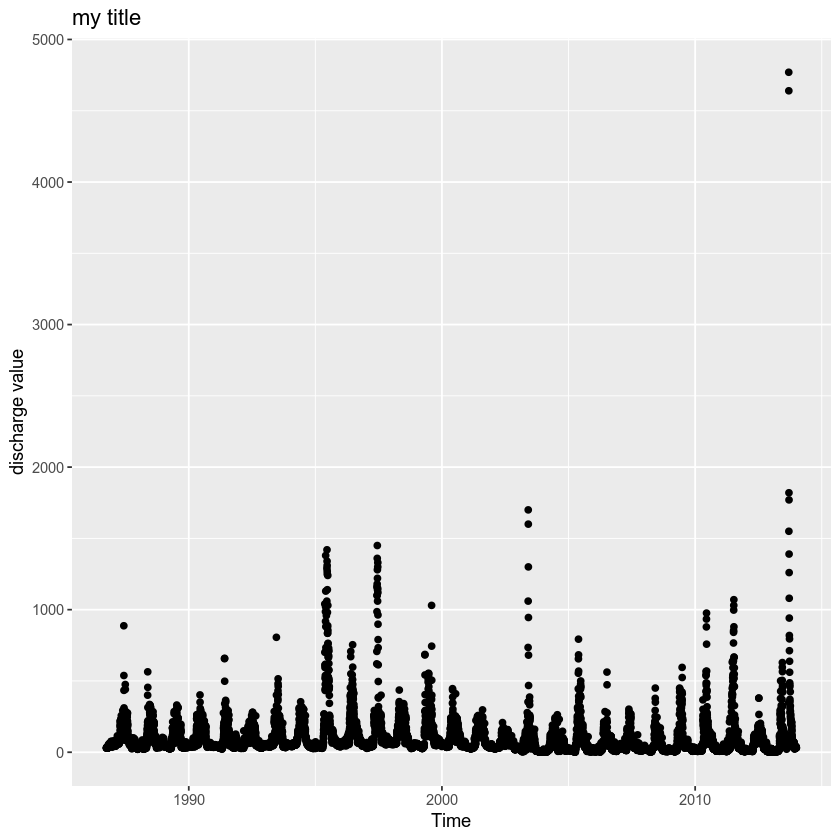# Lesson 6. Create Interactive Plots in R - Time Series & Scatterplots Using plotly and dygraphs

## Learning Objectives

After completing this tutorial, you will be able to:

• Create an interactive time series plot using plot.ly in R.
• Create an interactive time series plot using dygraphs in R.

## What You Need

You will need a computer with internet access to complete this lesson and the data for week 4 of the course.

In this lesson you will explore using 2 interactive tools to create interactive plots of your data:

1. plotly
2. dygraphs

First, you will load all of the needed libraries.

# install plotly from git - ropensci
#devtools::install_github('ropensci/plotly')

library(ggplot2)
library(xts)
library(dygraphs)
library(plotly)

options(stringsAsFactors = FALSE)


Next, let’s import some time series data

discharge_time <- read.csv("data/week-02/discharge/06730200-discharge-daily-1986-2013.csv")

# fix date using pipes
discharge_time <- discharge_time %>%
mutate(datetime = as.Date(datetime, format = "%m/%d/%y"))


You can plot the data using ggplot().

annual_precip <- ggplot(discharge_time, aes(x = datetime, y = disValue)) +
geom_point() +
labs(x = "Time",
y = "discharge value",
title = "my title")

annual_precip## Time Series - plotly

You can use plotly to create an interactive plot that you can use in the R console.

# create interactive plotly plot
ggplotly(annual_precip)



## Time Series - dygraph

Dygraph is a powerful and easy to use interactive time series plot generator. Below, notice how you can quickly create a dygraph interactive plot. The output format of the plot is html so it won’t work with a pdf rmd output but it will work with html!

# interactive time series
library(dygraphs)
# create time series objects (class xs)
library(xts)

# create time series object
discharge_timeSeries <- xts(x = discharge_time$disValue, order.by = discharge_time$datetime)



Then you can call the dygraph() function to create your interactive time-series plot.

# create a basic interactive element
interact_time <- dygraph(discharge_timeSeries)
interact_time

# create a basic interactive element
interact_time2 <- dygraph(discharge_timeSeries) %>% dyRangeSelector()
interact_time2